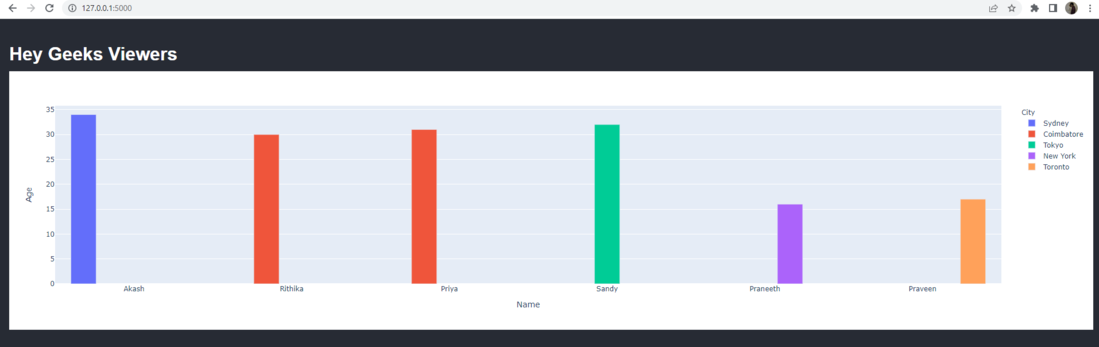Open in App
Not now

# Create a Bar Chart From a DataFrame with Plotly and Flask

• Last Updated : 23 Nov, 2022

Python has a number of powerful open-source libraries that make it an excellent language for Data Analysis and Web Development. One such library is Plotly, which makes it easy to create compelling data visualizations using web technologies. By combining Plotly with Flask, it is possible to create dynamic web pages that update automatically based on user interactions with the data. This article shows you how to use Plotly with Flask to generate a set of plots from the data stored in Pandas Dataframe.

Package Required

```pip install pandas
pip install plotly```

bar.html

In bar HTML page apply the necessary style elements for your page. Add headers and create the required div to display a chart, we use Javascript to invoke the Plotly chart using the JSON data sent by app.py as graphJSON parameter during the return.

## HTML

 `` `<``html``>` `<``style``>` `* {` `    ``-webkit-box-sizing: border-box;` `    ``box-sizing: border-box;` `}` `body {` `    ``background-color: #272b34;` `    ``font-family: 'Khula', sans-serif;` `    ``font-weight: 300;` `    ``color: white;` `    ``line-height: 1em;` `    ``margin: 0;` `  ``padding: 2em 1em;` `}`   `` ` ``<``body``>` `  ``<``h1``>Hey Geeks Viewers` `  ``<``div` `id``=``'chart'` `class``=``'chart'``”>` ``   `<``script` `src``=``'https://cdn.plot.ly/plotly-latest.min.js'``>` `<``script` `type``=``'text/javascript'``>` `  ``var graphs = {{graphJSON | safe}};` `  ``Plotly.plot('chart',graphs,{});` `` ``

app.py

Import the necessary modules. Have a dataset and design a home page route (Use default datasets available online or create our own). Dataset conversion to a Pandas Dataframe Now, we’ll use the Dataframe, Plotly, and px.bar to construct a bar chart (). Then, turn the figure into a JSON Object and send it using a render_template to the HTML page.

## Python3

 `# Import Required Modules` `from` `flask ``import` `Flask, render_template` `import` `pandas as pd` `import` `json` `import` `plotly` `import` `plotly.express as px`   `# Create Home Page Route` `app ``=` `Flask(__name__)`     `@app``.route(``'/'``)` `def` `bar_with_plotly():` `  `  `   ``# Students data available in a list of list` `    ``students ``=` `[[``'Akash'``, ``34``, ``'Sydney'``, ``'Australia'``],` `                ``[``'Rithika'``, ``30``, ``'Coimbatore'``, ``'India'``],` `                ``[``'Priya'``, ``31``, ``'Coimbatore'``, ``'India'``],` `                ``[``'Sandy'``, ``32``, ``'Tokyo'``, ``'Japan'``],` `                ``[``'Praneeth'``, ``16``, ``'New York'``, ``'US'``],` `                ``[``'Praveen'``, ``17``, ``'Toronto'``, ``'Canada'``]]` `    `  `    ``# Convert list to dataframe and assign column values` `    ``df ``=` `pd.DataFrame(students,` `                      ``columns``=``[``'Name'``, ``'Age'``, ``'City'``, ``'Country'``],` `                      ``index``=``[``'a'``, ``'b'``, ``'c'``, ``'d'``, ``'e'``, ``'f'``])` `    `  `    ``# Create Bar chart` `    ``fig ``=` `px.bar(df, x``=``'Name'``, y``=``'Age'``, color``=``'City'``, barmode``=``'group'``)` `    `  `    ``# Create graphJSON` `    ``graphJSON ``=` `json.dumps(fig, ``cls``=``plotly.utils.PlotlyJSONEncoder)` `    `  `    ``# Use render_template to pass graphJSON to html` `    ``return` `render_template(``'bar.html'``, graphJSON``=``graphJSON)`     `if` `__name__ ``=``=` `'__main__'``:` `    ``app.run(debug``=``True``)`

Output:

Run the command in the terminal.

`python app.py `http://127.0.0.1:5000/

My Personal Notes arrow_drop_up
Related Articles